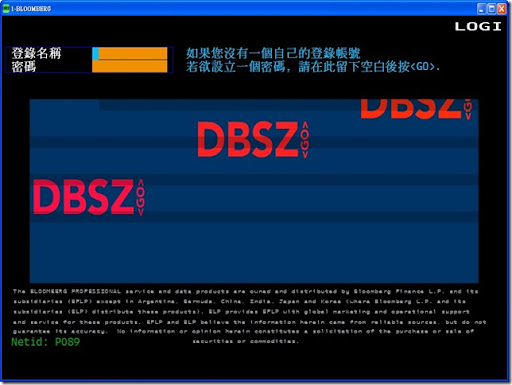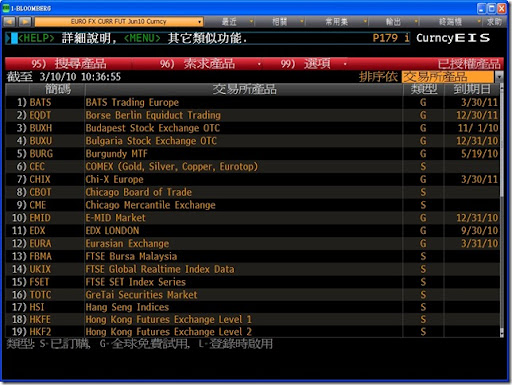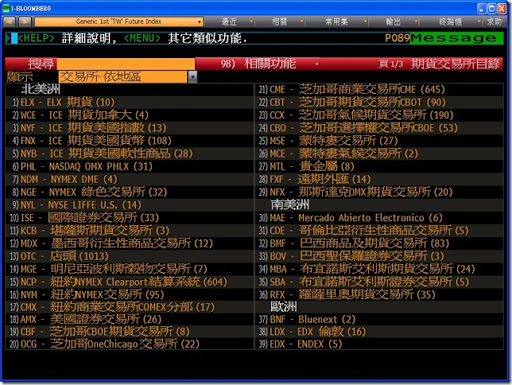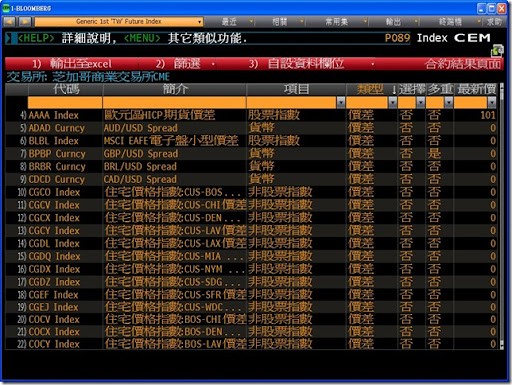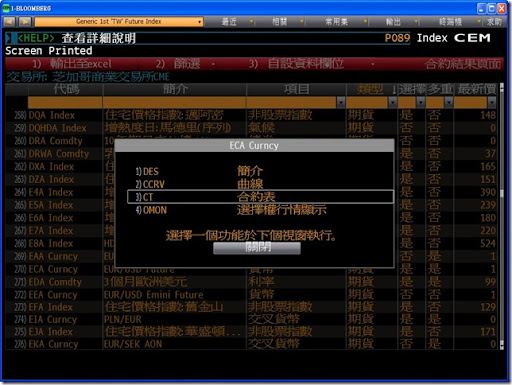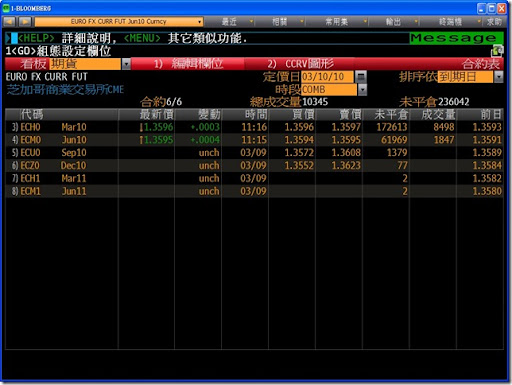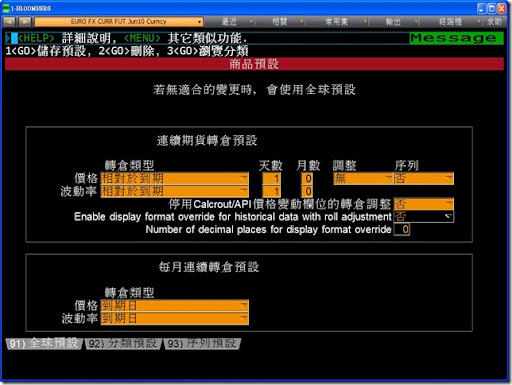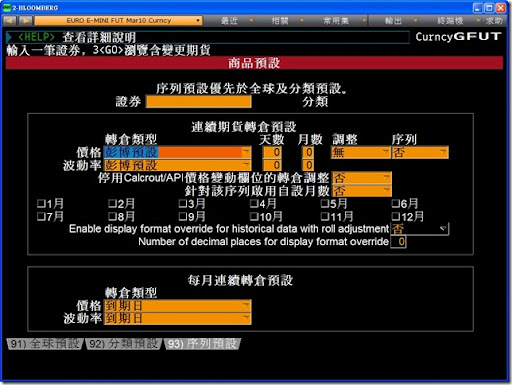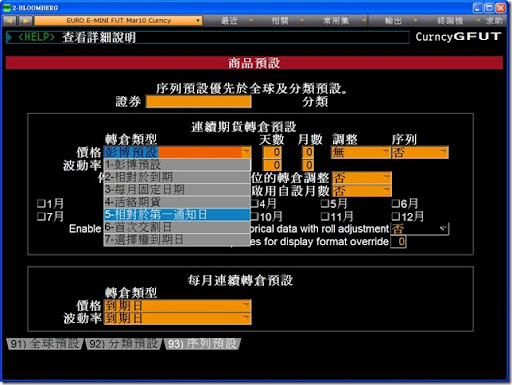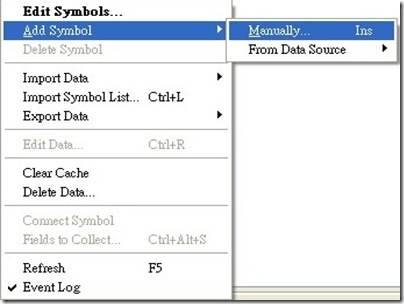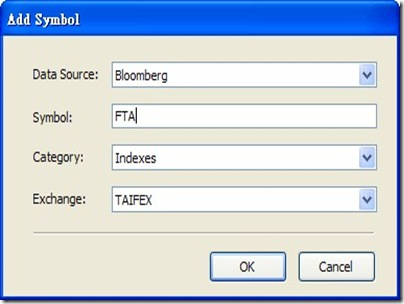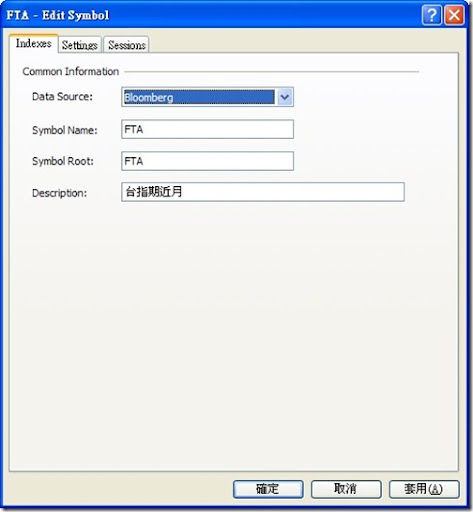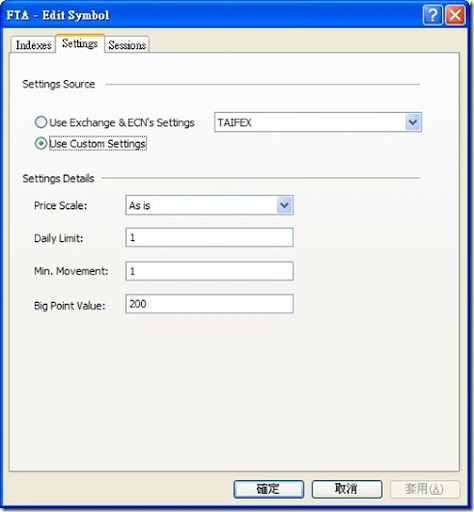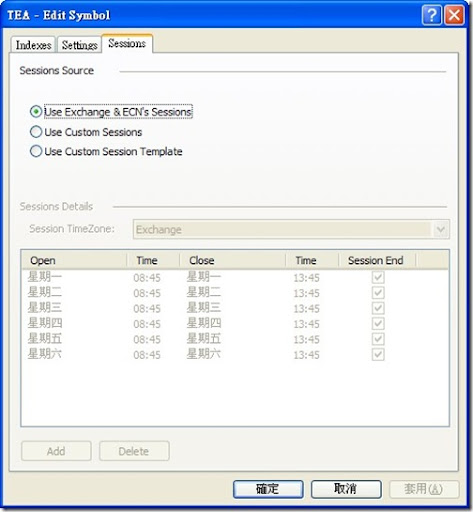2010年3月15日

利用 \$OHLCPeriodsAgo 修正 OpenD/HighD/LowD/CloseD 函數

1. \$OpenD
 inputs: PeriodsAgo( numericsimple ) ; variables: var1(0), var2(0), var3(0), var4(0) ; Value1 = \$OHLCPeriodsAgo( PeriodsAgo, var1, var2, var3, var4 ) ; \$OpenD = var1 ;

2. \$HighD
 inputs: PeriodsAgo( numericsimple ) ; variables: var1(0), var2(0), var3(0), var4(0) ; Value1 = \$OHLCPeriodsAgo( PeriodsAgo, var1, var2, var3, var4 ) ; \$OpenD = var2 ;

3. \$LowD
 inputs: PeriodsAgo( numericsimple ) ; variables: var1(0), var2(0), var3(0), var4(0) ; Value1 = \$OHLCPeriodsAgo( PeriodsAgo, var1, var2, var3, var4 ) ; \$LowD = var3 ;

4. \$CloseD
 inputs: PeriodsAgo( numericsimple ) ; variables: var1(0), var2(0), var3(0), var4(0) ; Value1 = \$OHLCPeriodsAgo( PeriodsAgo, var1, var2, var3, var4 ) ; \$LowD = var4 ;

修正 OHLCPeriodsAgo 函數-如何取得前N個交易時段之開/高/低/收

Multicharts 的 PowerLanguage Editor 內建了一個 OHLCPeriodsAgo 函數，可以取得 N天前的開/高/低/收價。但是這個函數是以日曆日作為交易時段之區分點，如果遇到跨天的交易時段（比如 CME Globex 交易之商品），算出來的結果會是錯誤的。

 Inputs:     PeriodsAgo(numericsimple),     oPeriodOpen(numericref),     oPeriodHigh(numericref),     oPeriodLow(numericref),     oPeriodClose(numericref) ; Variables: Count(0) ; Arrays: arr0[4,500](-1) ; // O/H/L/C IF (PeriodsAgo > 500 or BarType > 4) Then //Only keep 500 days of records     BEgin       oPeriodOpen = -1 ;       oPeriodHigh = -1 ;       oPeriodLow = -1 ;       oPeriodClose = -1 ;       \$OHLCPeriodsAgo = -1 ;     ENd ELSE     BEgin          For Value1 = 1 to SessionCount(0)          begin           If Value1 < SessionCount(0) then               Value2 = Value1 + 1           else             Value2 = 1;             Condition1 = (SessionEndDayMS(Value1) = DayofWeek(Date)) and (Time <= SessionEndTimeMS(Value1))                        AND                       (SessionStartDayMS(Value2) = DayofWeek(Date)) and (Time >= SessionStartTimeMS(Value2));           //Condition1 = (Time <= SessionEndTimeMS(Value1)) AND (Time >= SessionStartTimeMS(Value2));           if Condition1 then                                                   begin             Count = Count - 1 ;                                                                  if Count <= -1 then Count = 500;                                                         arr0[1,Count] = O ;                     arr0[2,Count] = H ;             arr0[3,Count] = L ;             arr0[4,Count] = C ;           end           else           begin              if H > arr0[2,Count] then arr0[2,Count] = H ;            if L < arr0[3,Count] then arr0[3,Count] = L ;            arr0[4,Count] = C;            if PeriodsAgo = 0 then arr0[4,Count] = C;           end ;       end;       oPeriodOpen = arr0[1,Mod(Count + PeriodsAgo, 501)] ;       oPeriodHigh = arr0[2,Mod(Count + PeriodsAgo, 501)] ;       oPeriodLow = arr0[3,Mod(Count + PeriodsAgo, 501)] ;        oPeriodClose = arr0[4,Mod(Count + PeriodsAgo, 501)] ;       \$OHLCPeriodsAgo = 1 ;     ENd; IF False THEN Value1 = \$OHLCPeriodsAgo ;

2010年3月10日

Multicharts QuoteManager 連接 Bloomberg 之設定方式